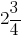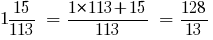# Convert mixed number to improper fraction

A result of conversion of mixed number to improper fraction is a fraction, the numerator of which is equal to the sum of the numerator of the fraction mixed with the product of the integer part by the denominator, and the denominator remains unchanged.

## To convert a mixed number to an improper fraction, you must:

1. multiply the integer portion by the denominator of the fractional part;
2. to the product add the numerator of the fractional part;
3. to record amount received by the numerator, and the denominator of the fractional part to leave without change.

### Examples of convert mixed numbers in improper fraction:

Example 1: Convert a mixed number to an improper fraction:Imagine an improper fraction number. Multiply it by the integer part by the denominator of the fractional part.

To the product add the numerator of the fractional part. Write the resulting sum is the numerator of the fraction, and the denominator of the fractional part stay the same, that isExample 2: to Convert a mixed number to an improper fraction:Tags:
Chapter:
Versions in other languages:
Share with friends: